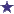# How Do I Calculate Days Supply in These Questions?

by Vanesa
(Austin, Texas)

1.)APAP with Codeine,240ml
5ml to 10ml q6h prn pain
Calculate the days supply.

2.)Ibuprofen 600mg tabs
i po QID
Calculate the days supply.

### Comments for How Do I Calculate Days Supply in These Questions?

Average RatingRatingby: David Answers: 1.) For the APAP with Codeine question, first you need to figure out the maximum amount of medication that could be used in one day. To find this, you see that 10ml every 6 hours is the maximum per day. (6/24 hours = 4) Then we multiply 10ml by 4 doses daily to come up with 40ml daily as a maximum dose. Divide the total amount the prescription calls for by fourty: 40/240 = 6. The prescription should have a days supply as 6 days. 2.)Ibuprofen 600mg tabs. Translate the sig: i po QID = 1 tab by mouth four times daily. The maximum amount per day is 4. However, we cannot find the days supply because there is no quantity given. A typical 30 days supply would require 120 tablets. (4x30=120) But, again, we are not given the amount.